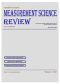MEASUREMENT SCIENCE REVIEW               Volume 4,  Section 1                       Main Page

 PAPER SECTION 1 Download and print the front cover  ->>Theoretical Problems of Measurement

1 - 10

Vojtko J.:

Abstract:  The paper describes the use of neural network as a component of measuring set for error reduction (nonlinearity). The scope of interest is elastomagnetic sensor used for measuring of massive pressure force (of range about 200 kN). Nonlinearity and hysteresis errors are main limitations of elastomagnetic sensor use. The hysteresis causes ambiguity of transfer characteristic and with it related impossibility of exact conversion of output sensor voltage into measured force. The function of the neural network is to regulate the basic metrological characteristics of sensor in order to achieve the smallest deviation from an ideal transfer characteristic. The assumption of the use of neural network as data-conditioning block is non-linear dependency of sensor output from input quantity.

11 - 19

Yong Y., Chongxun Z., Pan L.:

# A Novel Fuzzy C-Means Clustering Algorithm for Image Thresholding

Abstract:   Image thresholding has played an important role in image segmentation. In this paper, we present a novel spatially weighted fuzzy c-means (SWFCM) clustering algorithm for image thresholding. The algorithm is formulated by incorporating the spatial neighbourhood information into the standard FCM clustering algorithm. Two improved implementations of the k-nearest neighbour (k-NN) algorithm are introduced for calculating the weight in the SWFCM algorithm so as to improve the performance of image thresholding. To speed up the FCM algorithm, the iteration is carried out with the gray level histogram of image instead of the conventional whole data of image. The performance of the algorithm is compared with those of an existed fuzzy thresholding algorithm and widely applied between variance and entropy methods. Experimental results with synthetic and real images indicate the proposed approach is effective and efficient. In addition, due to the neighbourhood model, the proposed method is more tolerant to noise.